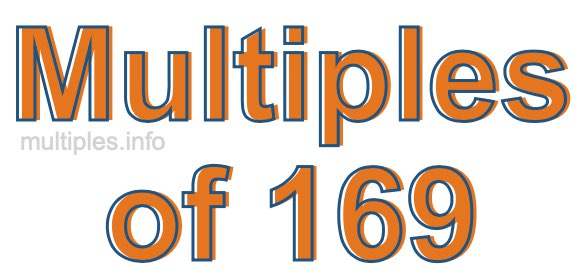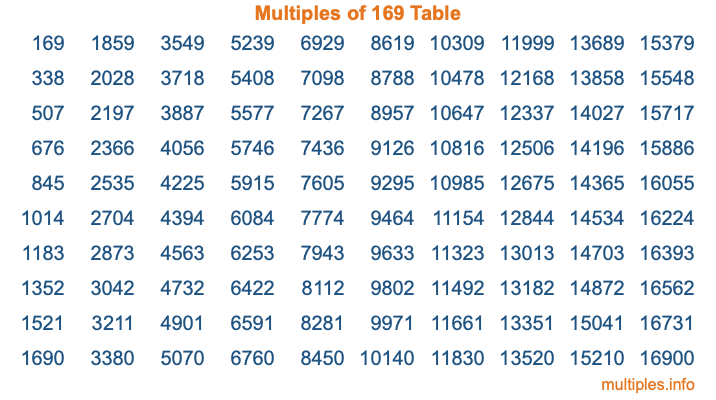Multiples of 169Welcome to the Multiples of 169 page. Here we will first teach you everything you will ever need to know about the multiples of 169, and then give you a study guide summary of everything we taught you to make sure you remember it all. Use this page to look up facts and learn information about the multiples of 169. This page will make you a multiples of one hundred sixty-nine expert!

Definition of Multiples of 169
Multiples of 169 are all the numbers that when divided by 169 equal an integer. Each of the multiples of 169 are called a multiple. A multiple of 169 is created by multiplying 169 by an integer.

Therefore, to create a list of multiples of 169, you start with 1 multiplied by 169, then 2 multiplied by 169, then 3 multiplied by 169, and so on for as long as you want. Thus, the list of the first five multiples of 169 is 169, 338, 507, 676, and 845. To see a larger list of multiples of 169, see the printable image of Multiples of 169 further down on this page. We also have a category where you can choose any nth multiple of 169.

Multiples of 169 Checker
The Multiples of 169 Checker below checks to see if any number of your choice is a multiple of 169. In other words, it checks to see if there is any number (integer) that when multiplied by 169 will equal your number. To do that, we divide your number by 169. If the the quotient is an integer, then your number is a multiple of 169.

Is  a multiple of 169?

Least Common Multiple of 169 and ...
A Least Common Multiple (LCM) is the lowest multiple that two or more numbers have in common. This is also called the smallest common multiple or lowest common multiple and is useful to know when you are adding our subtracting fractions. Enter one or more numbers below (169 is already entered) to find the LCM.

Check out our LCM Calculator if you need more details about the Least Common Multiple or if you need the LCM for different numbers for adding and subtraction fractions.

nth Multiple of 169
As we stated above, 169 is the first multiple of 169, 338 is the second multiple of 169, 507 is the third multiple of 169, and so on. Enter a number below to find the nth multiple of 169.

th multiple of 169

Multiples of 169 vs Factors of 169
169 is a multiple of 169 and a factor of 169, but that is where the similarities end. All postive multiples of 169 are 169 or greater than 169. All positive factors of 169 are 169 or less than 169.

Below is the beginning list of multiples of 169 and the factors of 169 so you can compare:

Multiples of 169: 169, 338, 507, 676, 845, etc.

Factors of 169: 1, 13, 169

As you can see, the multiples of 169 are all the numbers that you can divide by 169 to get a whole number. The factors of 169, on the other hand, are all the whole numbers that you can multiply by another whole number to get 169.

It's also interesting to note that if a number (x) is a factor of 169, then 169 will also be a multiple of that number (x).

Multiples of 169 vs Divisors of 169
The divisors of 169 are all the integers that 169 can be divided by evenly. Below is a list of the divisors of 169.

Divisors of 169: 1, 13, 169

The interesting thing to note here is that if you take any multiple of 169 and divide it by a divisor of 169, you will see that the quotient is an integer.

Multiples of 169 Table
Below is an image of the first 100 multiples of 169 in a table. The table is in chronological order, column by column. The first column has the first ten multiples of 169, the second column has the next ten multiples of 169, and so on.The Multiples of 169 Table is also referred to as the 169 Times Table or Times Table of 169. You are welcome to print out our table for your studies.

Negative Multiples of 169
Although not often discussed or needed in math, it is worth mentioning that you can make a list of negative multiples of 169 by multiplying 169 by -1, then by -2, then by -3, and so on, to get the following list of negative multiples of 169:

-169, -338, -507, -676, -845, etc.

Multiples of 169 Summary
Below is a summary of important Multiples of 169 facts that we have discussed on this page. To retain the knowledge on this page, we recommend that you read through the summary and explain to yourself or a study partner why they hold true.

There are an infinite number of multiples of 169.

A multiple of 169 divided by 169 will equal a whole number.

169 divided by a factor of 169 equals a divisor of 169.

The nth multiple of 169 is n times 169.

The largest factor of 169 is equal to the first positive multiple of 169.

169 is a multiple of every factor of 169.

169 is a multiple of 169.

A multiple of 169 divided by a divisor of 169 equals an integer.

169 divided by a divisor of 169 equals a factor of 169.

Any integer times 169 will equal a multiple of 169.

Multiples of a Number
Here you can get the multiples of another number, all with the same attention to detail as we did for multiples of 169 on this page.

Multiples of
Multiples of 170
Did you find our page about multiples of one hundred sixty-nine educational? Do you want more knowledge? Check out the multiples of the next number on our list!